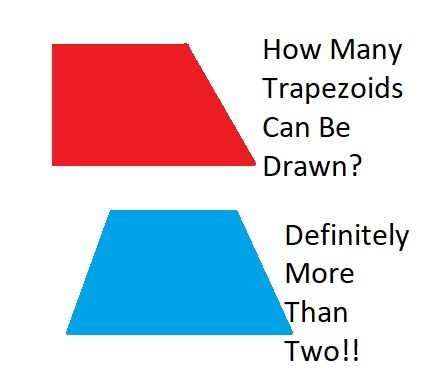# 37 and How Many Trapezoids I Can DrawA trapezoid is often defined for young students as a four-sided shape with EXACTLY two parallel sides. Once a person studies higher level math, the definition changes: A trapezoid is a four-sided shape with AT LEAST two parallel sides. How many different kinds of trapezoids can a person draw? It depends on which definition you use. If you use the second definition, you can also include parallelograms, rectangles, rhombuses, and squares. Either definition will allow the standard isosceles trapezoid and several others. But how many? Whichever definition you use, figuring out how many different ones can be drawn is a nice puzzle to solve. NebusResearch’s blog post does a nice job explaining the different ones, and it even came up with ones I hadn’t considered!

Now I’ll share some facts about the number 37:

• 37 is a prime number.
• Prime factorization: 37 is prime.
• The exponent of prime number 37 is 1. Adding 1 to that exponent we get (1 + 1) = 2. Therefore 37 has exactly 2 factors.
• Factors of 37: 1, 37
• Factor pairs: 37 = 1 x 37
• 37 has no square factors that allow its square root to be simplified. √37 ≈ 6.08276How do we know that 37 is a prime number? If 37 were not a prime number, then it would be divisible by at least one prime number less than or equal to √37 ≈ 6.1. Since 37 cannot be divided evenly by 2, 3, or 5, we know that 37 is a prime number.

37 is never a clue in the FIND THE FACTORS puzzles.

1.Joseph Nebus